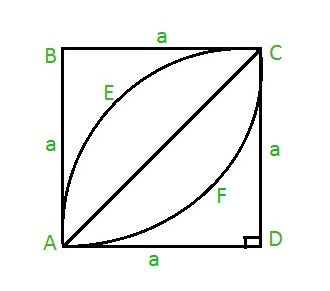GeeksforGeeks App
Open AppBrowser
Continue

# Area of a leaf inside a square

Given an integer a as the side of the square ABCD. The task is to find the area of the leaf AECFA inside the square as shown below:Examples:

Input: a = 7
Output: 28

Input: a = 21
Output: 252

Approach: To calculate the area of the leaf, first find the area of the half leaf AECA, which can be given as:

Area of half leaf = Area of quadrant AECDA – Area of right triangle ACD
Thus, Area of half leaf = ( PI * a * a / 4 ) – a * a / 2 where PI = 22 / 7 and a is the side of the square.
Hence, the area of full leaf will be ( PI * a * a / 2 ) – a * a
On taking a * a common we get, Area of leaf = a * a ( PI / 2 – 1 )

Below is the implementation of the above approach:

## C

 `// C program to find the area of``// leaf inside a square``#include ``#define PI 3.14159265` `// Function to find area of``// leaf``float` `area_leaf(``float` `a)``{``    ``return` `(a * a * (PI / 2 - 1));``}` `// Driver code``int` `main()``{``    ``float` `a = 7;``    ``printf``(``"%f"``,``           ``area_leaf(a));``    ``return` `0;``}`

## Java

 `// Java code to find the area of``// leaf inside a square``import` `java.lang.*;` `class` `GFG {` `    ``static` `double` `PI = ``3.14159265``;` `    ``// Function to find the area of``    ``// leaf``    ``public` `static` `double` `area_leaf(``double` `a)``    ``{``        ``return` `(a * a * (PI / ``2` `- ``1``));``    ``}` `    ``// Driver code``    ``public` `static` `void` `main(String[] args)``    ``{``        ``double` `a = ``7``;``        ``System.out.println(area_leaf(a));``    ``}``}`

## Python3

 `# Python3 code to find the area of leaf``# inside a square``PI ``=` `3.14159265``    ` `# Function to find the area of``# leaf``def` `area_leaf( a ):``    ``return` `( a ``*` `a ``*` `( PI ``/` `2` `-` `1` `) )``    ` `# Driver code``a ``=` `7``print``(area_leaf( a ))`

## C#

 `// C# code to find the area of``// leaf``// inside square``using` `System;` `class` `GFG {``    ``static` `double` `PI = 3.14159265;` `    ``// Function to find the area of``    ``// leaf``    ``public` `static` `double` `area_leaf(``double` `a)``    ``{``        ``return` `(a * a * (PI / 2 - 1));``    ``}` `    ``// Driver code``    ``public` `static` `void` `Main()``    ``{``        ``double` `a = 7;``        ``Console.Write(area_leaf(a));``    ``}``}`

## PHP

 ``

## Javascript

 ``

Output:

`28`

Time complexity: O(1), since there is no loop or recursion.

Auxiliary Space: O(1), since no extra space has been taken.

My Personal Notes arrow_drop_up